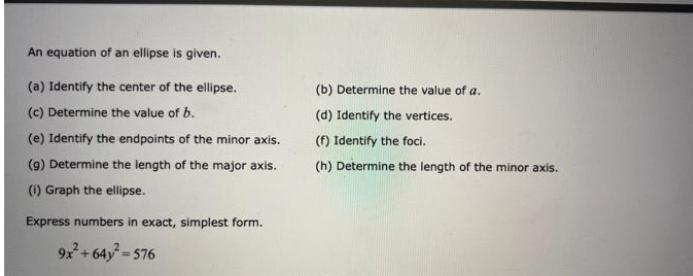Question:

# An equation of an ellipse is given. (a) Identify the center of the ellipse. (b) Determine the value of a. (c) Determine the value of b. (d) Identify the vertices. (e) Identify the endpoints of the miAn equation of an ellipse is given. (a) Identify the center of the ellipse. (b) Determine the value of a. (c) Determine the value of b. (d) Identify the vertices. (e) Identify the endpoints of the minor axis. (f) Identify the foci. (g) Determine the length of the major axis. (h) Determine the length of the minor axis. (i) Graph the ellipse. Express numbers in exact, simplest form. 9x^2 + 64y^2 = 576